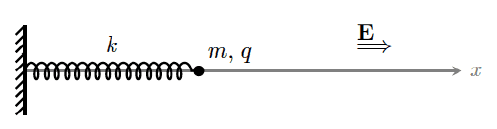# Hamiltonian of this system?

• pepediaz
pepediaz
Homework Statement
Not homework exactly :-[
Relevant Equations
H = T + U (I posted an attempt to the solution below)
Let a mass m charged with q, attached to a spring with constant factor k = mω ^2 in an electric field E(t) = E0(t/τ) x since t=0.
(Equilibrium position is x0 and the deformation obeys ξ = x - x0)

What would the hamiltonian and motion equations be in t ≥ 0, in terms of m and ω?? Despise magnetic field.Last edited:

Mentor
Moderator's note: Moved to homework forum.

Mentor

pepediaz
2) It is correct to assume time symmetry and so equate this "energy function" to the canonic hamiltonian?
3) Motion equations come from hamiltonian or from lagrangian?

Thanks

Last edited:
Mentor
pepediaz
But it is okay to have the development in the picture??

Mentor
But it is okay to have the development in the picture??

No. As I said, you need to use the PF LaTeX feature for equations. I gave you the link to the help page for it.

pepediaz
Is it okay to do it in RuBbEr??

Period

Mentor
Is it okay to do it in RuBbEr??

I have no idea what that is, but I assume it's not LaTeX. LaTeX is the only option you have here for posting equations. If you cannot or will not use LaTeX, we cannot help you.